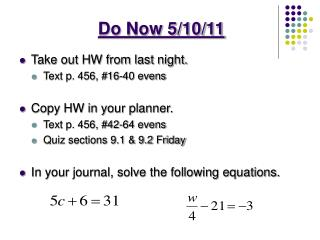DownloadDownload PresentationDo Now 5/10/11

# Do Now 5/10/11

Download Presentation## Do Now 5/10/11

- - - - - - - - - - - - - - - - - - - - - - - - - - - E N D - - - - - - - - - - - - - - - - - - - - - - - - - - -
##### Presentation Transcript

1. Do Now 5/10/11 • Take out HW from last night. • Text p. 456, #16-40 evens • Copy HW in your planner. • Text p. 456, #42-64 evens • Quiz sections 9.1 & 9.2 Friday • In your journal, solve the following equations.

2. 16) ±5 18) ±9 20) ±32 22) ±40 24) 7 feet 26) -11 28) ±4 30) ±16 32) -3 34) -3.2 36) 10.5 38) 36.4 40) 2.6 Homework Text p. 456, #16-40 evens

3. Objective • SWBAT simplify expressions with radicals and solve equations using square roots

4. Section 9.1 “Square Roots” If b² = a then b is the square root of a. TheSQUARE ROOTof a number is denoted by the symbol , which is called aradical. radicand

5. Evaluate the Expression √ a – b a = 48, b = 12 √ 48 – 12 PEMDAS √ 36 6

6. Evaluate the Expression -3√ ab a = 48, b = 12 -3√ (48)(12) PEMDAS -3√ 576 -3(24) -72

7. Try it out…Evaluate the Expression a = 11 b = 5 a = 3 b = 7

8. Solve Equations Using Square Roots To solve an equation with an x², you need to ISOLATE the x² on one side and then take the square root of both sides of the equation. If then If then

9. Equation Remember this? 2 s + 8 = 20 Left side Right side ‘s’ has to be 6 in order to make the equation balanced. Like a scale, the left side and right side must be the same in order to be balanced!

10. Equation- Remember this? • mathematical sentence with an equal sign Like a scale, both sides must be EQUAL in order to be balanced.

11. Solving Equations with x²… Isolate the variable! Get ‘x²’ by itself. x² + 5 = 21 To get the ‘x²’ by itself first get rid of “adding 5.” • 5 • 5 Do the opposite. “Subtract 5.” Whatever you do to one side of the equation you must do the other side. x² = 16 √ √ To get the ‘x’ by itself get rid of the “square” by using the square root. x = ±4

12. Solving Equations with x²… 3x² - 7 = 18 +7 +7 3x² = 25 3 3 √ x² = 8.3 √ x = ±2.9

13. Try it out…Round your solutions to the nearest tenth

14. Homework Text p. 456, #42-64 evens